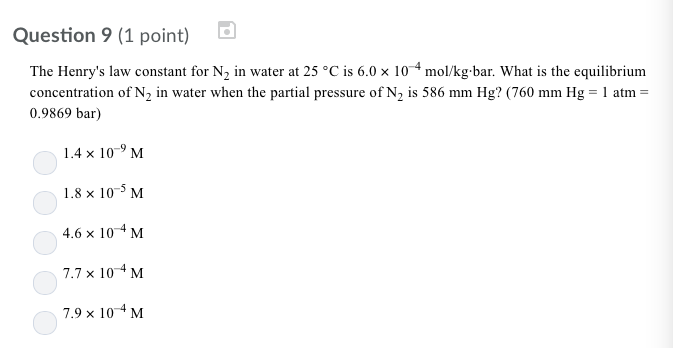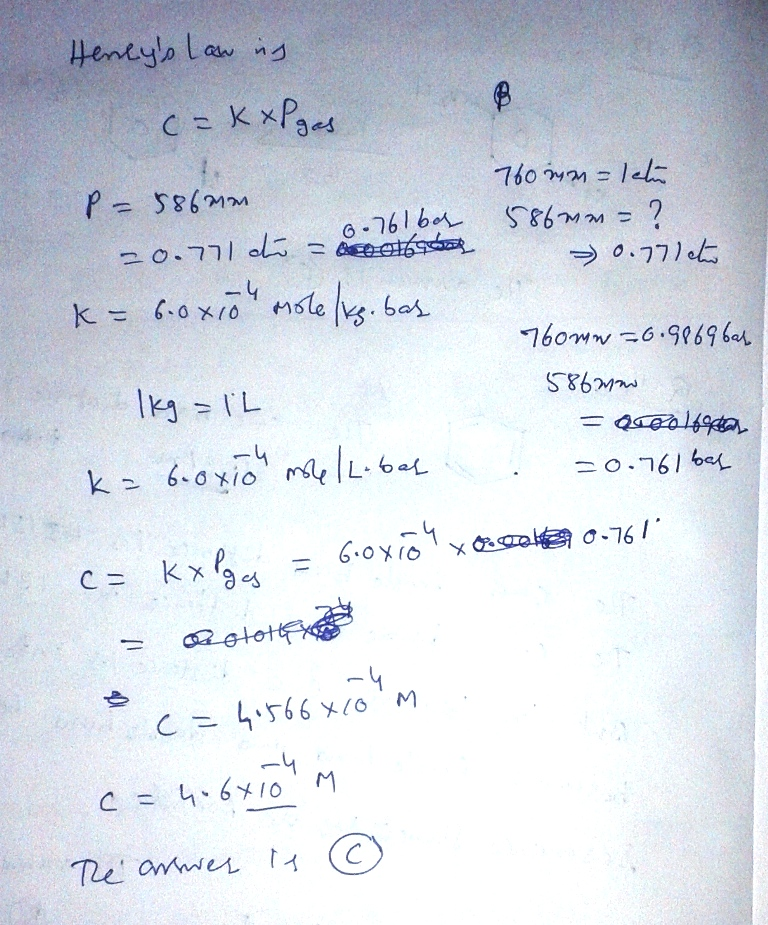# Question & Answer: The Henry's law constant for N_2 in water at 25 degree C is 60 times 10^-4 mol/kg middot…..The Henry’s law constant for N_2 in water at 25 degree C is 60 times 10^-4 mol/kg middot bar. What is the equilibrium concentration of N_2 in water when the partial pressure of N_2 is 586 mm Hg? (760 mm Hg = 1 atm = 0.9869 bar) 1.4 times 10^-9 M 1.8 times 10^-5 M 4.6 times 10^-4 M 7.7 times 10^-4 M 7.9 times 10^-4 M